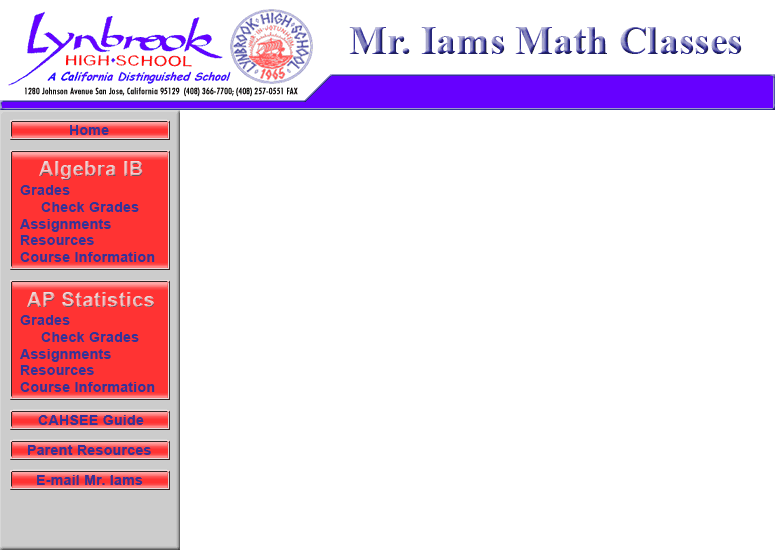Key Concepts: Algebra I

Algebra 2 / Trigonometry Content Standards:
CA Common Core Standards for Algebra 2
Previous Content Standards

Ask Dr. Math - good for obtaining an alternate explanation of almost any math concept.

Purple Math - provides detailed lessons and good links.
Linear Equation Solver - a great tool from DiscoverySchool.com
You can use it to solve almost any linear equation. Yes, it does homework problems too!
Calculator.com - Free Scientific Calculator (But beware lots of junk links and pop ups.
Do not click any of the links or enter any personal information!)
Graphing Calculator - a great tool for graphing linear and quadratic equations and finding the solution to a system of linear equations using the graphing method. When using this program be sure to have your equations solved for one variable (usually y) and set the graph appropriately (Xmin Xmax and Ymin Ymax). Also be sure to use ^ for squared, x^2.
Systems of Equations Sovler - another great too from DiscoverySchool.com. You can use it to solve almost any system of two equations with two variables. Yes, it does homework problems too!